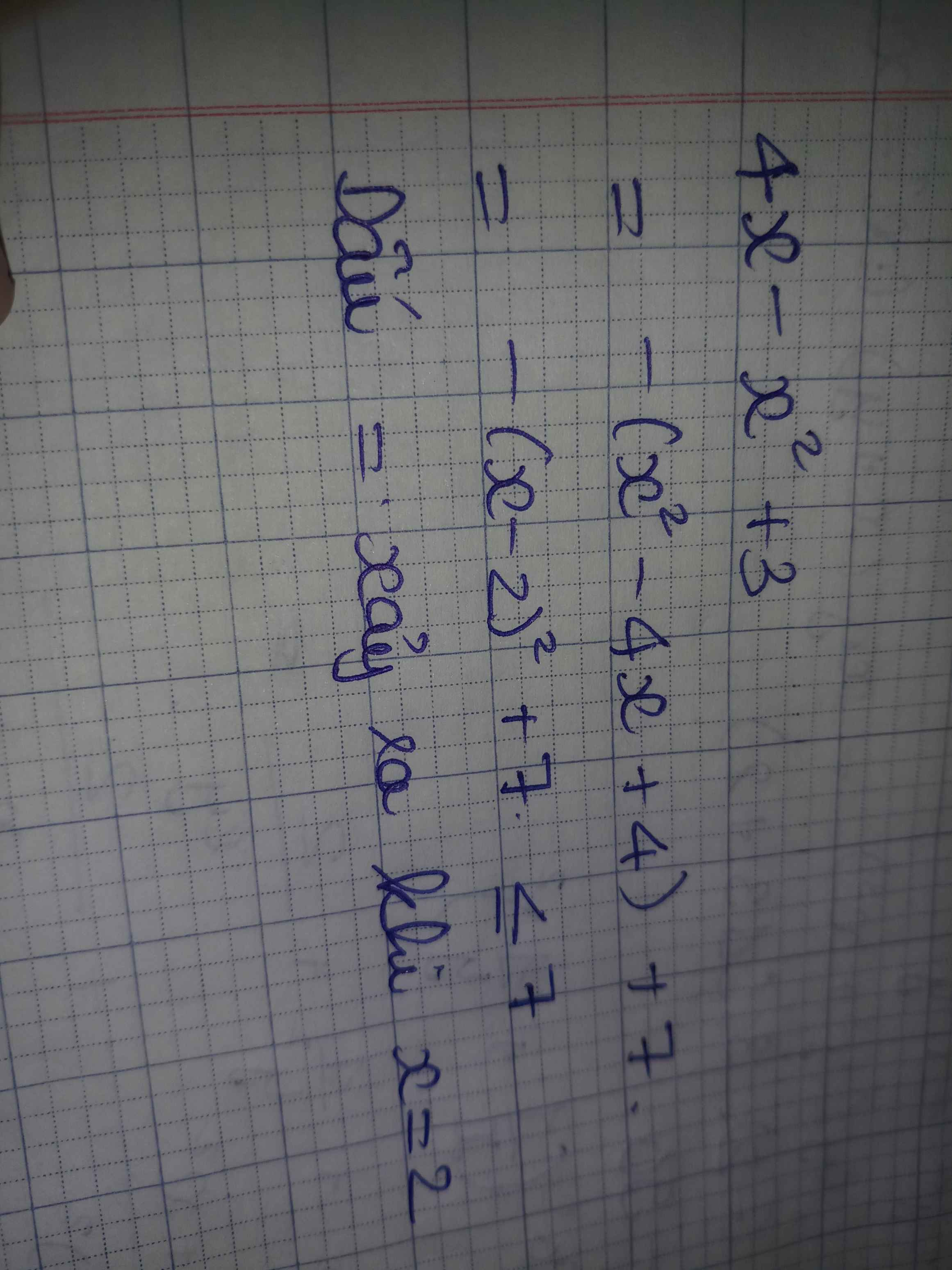2 phút trước

Ta có: $-x^2+4x+3=-\left(x^2-4x+4\right)+7=-\left(x-2\right)^2+7\le7\forall x\in R$

⇒ max y = 7 tại x = 2

Bình luận (0)Vài giây trướcBình luận (0)